# Making Hilbert Curve

Author: Zuguang Gu ( z.gu@dkfz.de )

Date: 2015-10-14

Hilbert curve is a type of space-filling curves that fold one dimensional axis into a two dimensional space, but with still keeping the locality. It has advantages to visualize data with long axis in following two aspects:

1. greatly improve resolution for the visualization;
2. easy to visualize clusters because generally data points in the cluster will also be close in the Hilbert curve.

This package aims to provide an easy and flexible way to visualize data through Hilbert curve. The implementation and example figures are based on following sources:

suppressPackageStartupMessages(library(HilbertCurve))
library(circlize)
set.seed(12345)


Following shows Hilbert curve with level 2, 3, 4, 5: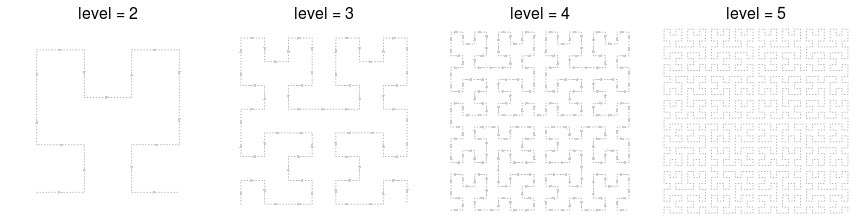Following heatmap shows distance between elements in the Hilbert curve. From left to right and from top to bottom, the order is the natural order of data points and colors correspond to the distance for pair distance in the Hilbert curve. Basically, if data points are close in the one dimensional axis, they also have small distance in the Hilbert curve (regions around diagonals)

library(HilbertVis)
pos = HilbertVis::hilbertCurve(5)
mat = as.matrix(dist(pos))
library(ComplexHeatmap)

ht = Heatmap(mat, name = "dist", cluster_rows = FALSE, cluster_columns = FALSE,
show_row_names = FALSE, show_column_names = FALSE,
heatmap_legend_param = list(title = "euclidean_dist"))
draw(ht, padding = unit(c(5, 5, 5, 2), "mm"))
decorate_heatmap_body("dist", {
grid.segments(c(0.25, 0.5, 0.75, 0, 0, 0), c(0, 0, 0, 0.25, 0.5, 0.75),
c(0.25, 0.5, 0.75, 1, 1, 1), c(1, 1, 1, 0.25, 0.5, 0.75), gp = gpar(lty = 2))
grid.text(rev(c(256, 512, 768, 1024)), 0, c(0, 256, 512, 768)/1024, just = "bottom",
rot = 90, gp = gpar(fontsize = 10))
grid.text(c(1, 256, 512, 768, 1024), c(1, 256, 512, 768, 1024)/1024, 1, just = "bottom",
gp = gpar(fontsize = 10))
})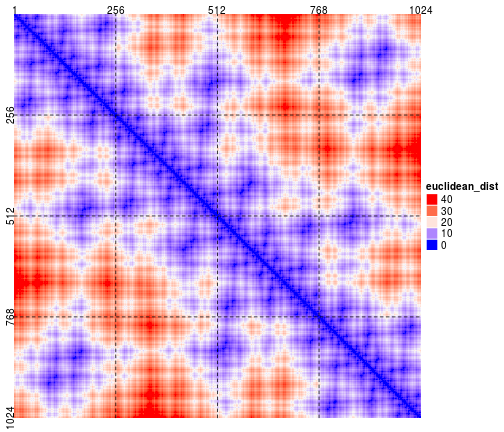## Basic settings

Generally, customizing a Hilbert curve follows following steps:

hc = HilbertCurve(...)
hc_points(hc, ...)
hc_rect(hc, ...)
hc_segments(hc, ...)
hc_text(hc, ...)


HilbertCurve() is a constructor function and initializes the Hilbert curve. Here the start and end position that correspond to the two ends of the Hilbert curve are specified as well as the level of the Hilbert curve. The function returns a HilbertCurve class instance and it can be used to add more graphics later.

hc = HilbertCurve(1, 100, level = 4, reference = TRUE)


The curve can be though as a folded axis. When the coordinate for this folded axis is initialized, low-level graphics can be added with specifying the positions.

Before we demonstrate low-level functions, first we generate a random regions by IRanges package.

x = sort(sample(100, 20))
s = x[1:10*2 - 1]
e = x[1:10*2]
ir = IRanges(s, e)
ir

## IRanges of length 10
##      start end width
##       1   4     4
##      14  15     2
##      16  31    16
##      33  34     2
##      40  44     5
##      48  65    18
##      67  73     7
##      75  78     4
##      86  87     2
##     91  97     7


### Points

There are two modes for adding points. By default, every segment in the Hilbert curve are segmented into several small segments and a circle is plotted at every small segments.

hc = HilbertCurve(1, 100, level = 4, reference = TRUE)
hc_points(hc, ir)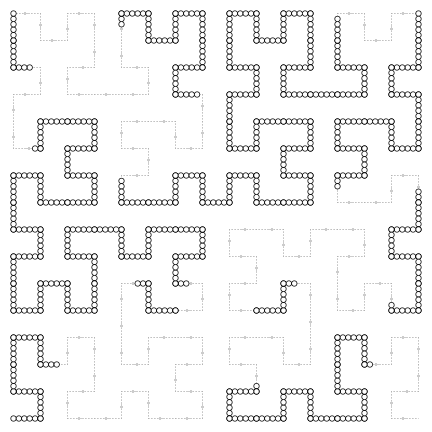The number of circles in the small segment can be controlled by np and graphical parameters can be set by gp. Note under this mode, the size of points can only be changed by np argument. This mode is useful if you have long regions.

hc = HilbertCurve(1, 100, level = 4, reference = TRUE)
hc_points(hc, ir, np = 3, gp = gpar(fill = rand_color(length(ir))))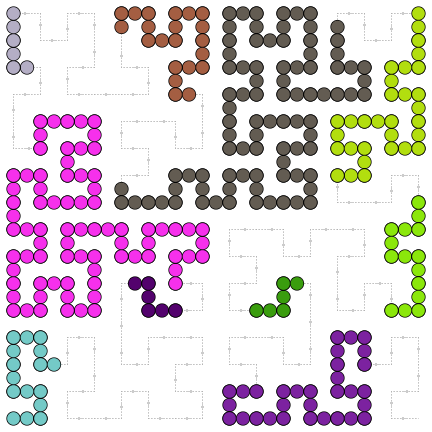Here such circles are not real points in R, they are polygons actually. There are some pre-defined shapes that you can choose from: “circle”, “square”, “triangle”, “hexagon”, “star”.

If np is set less than 2 or NULL, the points will be plotted at the center of every interval in ir. In this case, size argument is used to control the size of the points. This mode is useful if you have a lot of small regions.

hc = HilbertCurve(1, 100, level = 4, reference = TRUE)
hc_points(hc, ir, np = NULL, size = unit(runif(length(ir)), "cm"), pch = 16)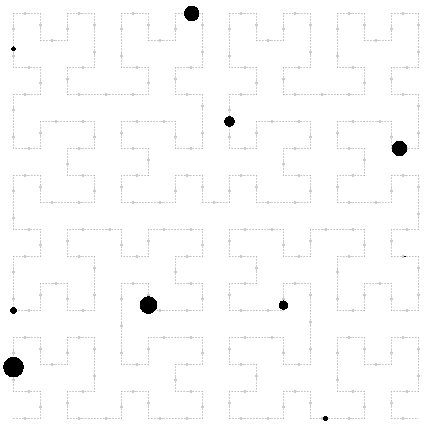### Segments

hc = HilbertCurve(1, 100, level = 4, reference = TRUE)
hc_segments(hc, ir)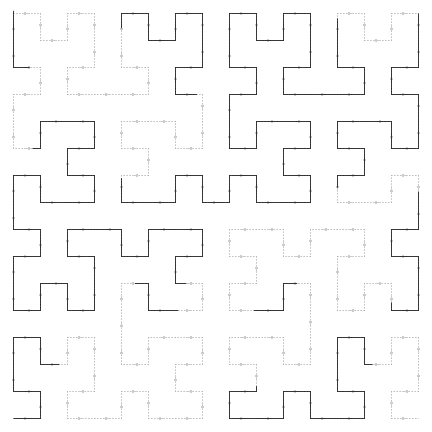### Rectangles

Adding rectangles is straightforward. Note You cannot set the width or height of the rectangles. Rectangles are always located at the turning points and have width or height equal to the length of the segments.

hc = HilbertCurve(1, 100, level = 4, reference = TRUE)
hc_rect(hc, ir)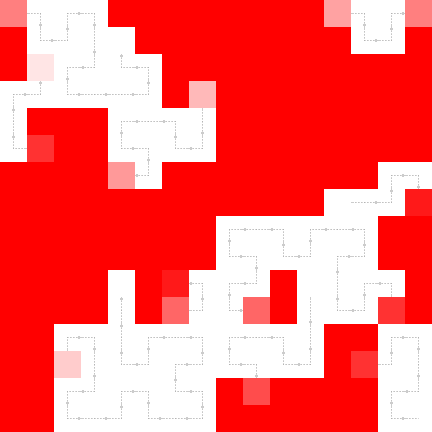As you can see some rectangles are not full red. It is because these segments in the curve do not full cover regions in ir, Averaging is applied in such segments. Actually it is also applicable for the 'segmented circle' mode for hc_points.

Graphical values that correspond to ir are always represented as numeric values, such as size of points, width of lines or even colors which can be converted to numeric RGB values. If a segment in the Hilbert curve can not full overlap with some intervals in ir, these numeric parameters can be averaged.

There are three different modes for averaging which are controlled by mean_mode argument. Following illustrates different settings for mean_mode:

      100     80    60     values in ir (e.g. red compoment for colors)
++++++   +++   +++++   ir
================     window (width = 16)
4      3     3      overlap

absolute: (100 + 80 + 60)/3
weighted: (100*4 + 80*3 + 60*3)/(4 + 3 + 3)
w0:       (100*4 + 80*3 + 60*3)/16


So use of the mode depends on specific scenario. For example, if ir corresponds to positions of genes, then the mode of w0 is perhaps a good choise. If ir corresponds to positions of CpG sites which is has width of 1 and most of the time is sparse in genomic windows, then absolute is a correct choice.

### Text

Adding texts is straightforward. Note text is added at the center of each interval in ir.

hc = HilbertCurve(1, 100, level = 4, reference = TRUE)
labels = sample(letters, length(ir), replace = TRUE)
hc_text(hc, ir, labels = labels)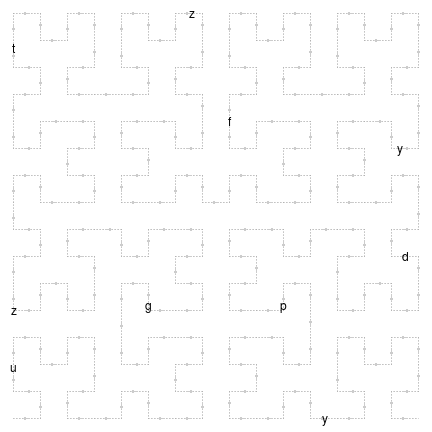### Combine low-level functions

With combination of these basic graphic functions, complicated graphics can be easily made:

hc = HilbertCurve(1, 100, level = 4)
hc_segments(hc, IRanges(1, 100))   # background line
hc_rect(hc, ir)
hc_points(hc, ir, np = 3)
hc_text(hc, ir, labels = labels, gp = gpar(fontsize = 16, col = "blue"))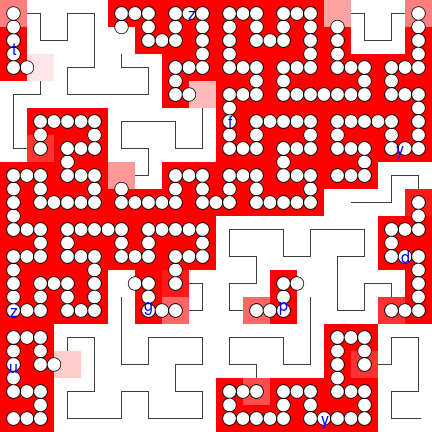## Non-integer positions

It doesn't matter if your positions are integers or not. Internally, rounding and zooming are applied to ensure the accuracy.

Since the positions are not integers, you can specify the positions by x1 and x2. All low-level graphical funtions accept x1 and x2.

hc = HilbertCurve(0.1, 0.8, level = 4, reference = TRUE)
hc_points(hc, x1 = c(0.15, 0.55), x2 = c(0.25, 0.65))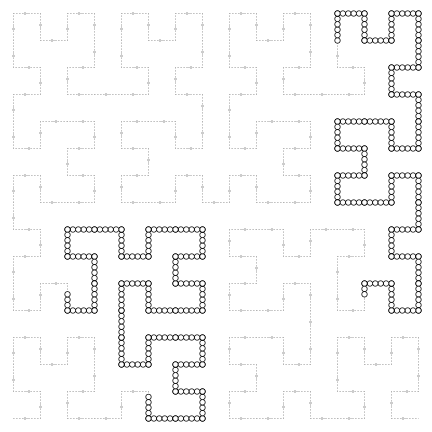Title are allowed by setting title argument. Legend can be passed to legend argument in HilbertCurve() as a grob object or a list of grob objects. ColorMapping class in ComplexHeatmap package or legendGrob() in grid package can be used to create a legend grob. Or you can consider to use frameGrob() and packGrob() to build a legend from ground.

Because width and height are enforced to be equal for the Hilbert curve, sometimes you may see blank spaces around the curve.

value = runif(length(ir))
col_fun = colorRamp2(c(0, 1), c("white", "red"))
cm = ColorMapping(col_fun = col_fun)
legend = color_mapping_legend(cm, plot = FALSE, title = "value")
hc = HilbertCurve(1, 100, reference = TRUE, title = "points", legend = legend)
hc_points(hc, ir, np = 3, gp = gpar(fill = col_fun(value)))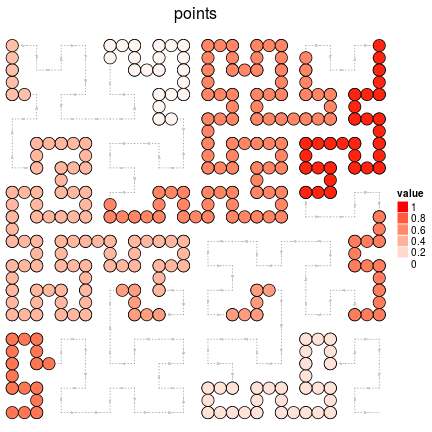## Pixel mode

When the level is high (e.g. > 10), the whole 2D space will be almost completely filled by the curve and it is impossible to add or visualize e.g. points on the curve. In this case, the 'pixel' mode visualizes each tiny 'segment' as a pixel and maps values to colors. So the Hilbert curve with level 11 will generate a PNG figure with 2048x2048 resolution. This is extremely useful for visualize genomic data. E.g. If we make a Hilbert curve for human chromosome 1 with level 11, then each pixel can represent 60bp (249250621/2048/2048) which is of very high resolution.

Under 'pixel' mode, if the current device is an interactive deivce, every time a new layer is added, the image will be add to the interactive device as a rastered image.

hc = HilbertCurve(1, 100, level = 9, mode = "pixel")
hc_layer(hc, ir)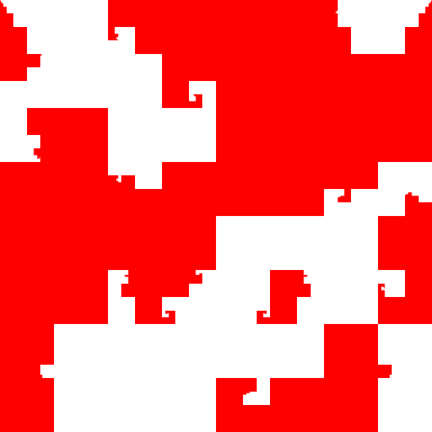Since you can only use color to map to other values, there is only one graphical setting col. You can add more than one layers, just remember to set transparent colors. And of course you can add title and legend to the plot. Grid lines can be added to the plot for better distinguish blocks in the Hilbert curve.

hc = HilbertCurve(1, 100, level = 9, mode = "pixel", title = "pixel mode", legend = legend)
hc_layer(hc, ir, col = col_fun(value), grid_line = 2)
hc_layer(hc, x1 = 75, x2 = 85, col = "#0000FF10")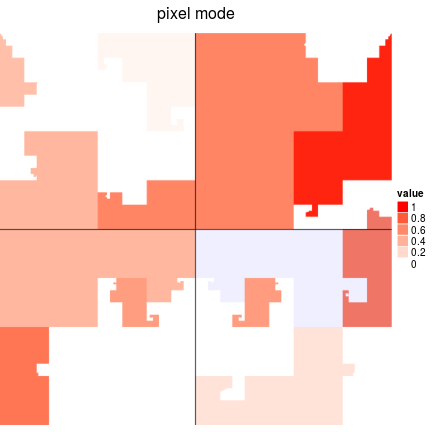The Hilbert curve can be save as PNG figure by hc_png(), with resolution 2^level x 2^level.

hc_png(hc, file = "test.png")


## Examples

Visualize rainbow colors:

col = rainbow(100)
hc = HilbertCurve(1, 100, level = 5)
ir = IRanges(start = 1:99, end = 2:100)
hc_points(hc, ir, np = 4, gp = gpar(col = col, fill = col))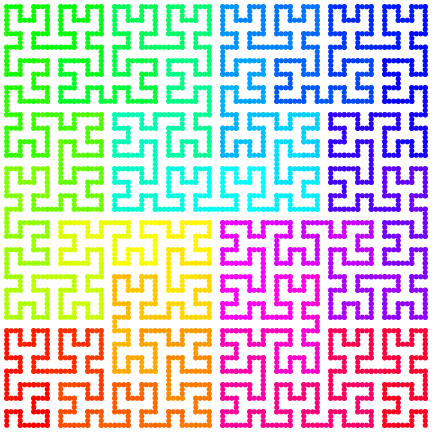Genes on chromosome 1 (RefSeq genes for human, hg19). Here random colors are used to represent to different genes.

chr1_len = 249250621
hc = HilbertCurve(1, chr1_len, level = 5)
hc_segments(hc, IRanges(start = 1, end = chr1_len), gp = gpar(col = "grey"))
hc_segments(hc, ranges(g), gp = gpar(lwd = unit(6, "mm"), col = rand_color(length(g))))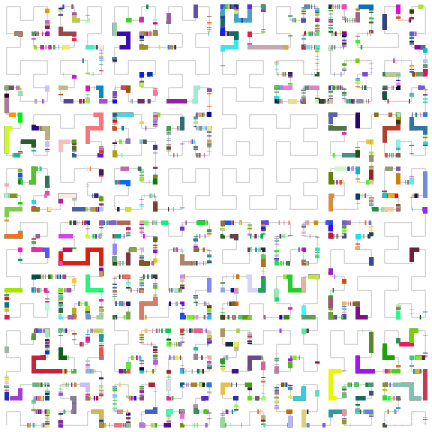Following two figures compare sequence conservations. Data are from here.

load(paste0(system.file("extdata", package = "HilbertCurve"), "/mouse_net.RData"))
hc = HilbertCurve(1, chr1_len, level = 6)
ir1 = ranges(mouse)
ir2 = setdiff(IRanges(1, chr1_len), ir1)
ir = c(ir1, ir2)
col = c(rep("red", length(ir1)), rep("yellow", length(ir2)))
hc_points(hc, ir, np = 3, gp = gpar(col = col, fill = col))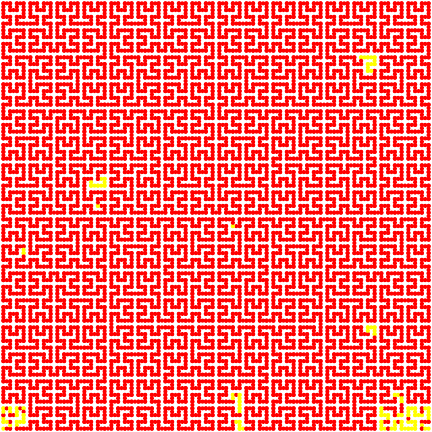load(paste0(system.file("extdata", package = "HilbertCurve"), "/zebrafish_net.RData"))
hc = HilbertCurve(1, chr1_len, level = 6)
ir1 = ranges(zebrafish)
ir2 = setdiff(IRanges(1, chr1_len), ir1)
ir = c(ir1, ir2)
col = c(rep("red", length(ir1)), rep("yellow", length(ir2)))
hc_points(hc, ir, np = 3, gp = gpar(col = col, fill = col))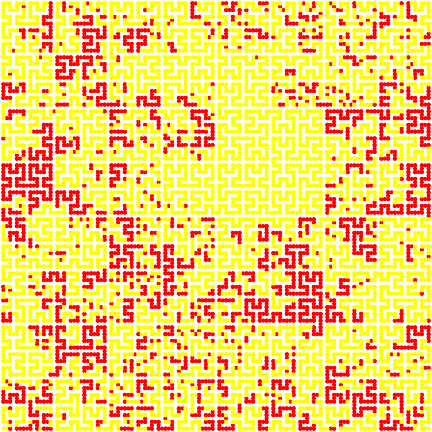GC percent on chromosome 1, under “normal” mode:

df = read.table(pipe("awk '\$1==\"chr1\"' /path-to/hg19_gc_percent_window1000.bed"))
col_fun = colorRamp2(c(0, 500, 1000), c("green", "#FFFFCC", "red"))
png("gc_percent_chr1_points.png", width = 500, height = 500)
hc = HilbertCurve(1, chr1_len, level = 6)
hc_points(hc, IRanges(df[], df[]), np = 3, gp = gpar(fill = col_fun(df[]), col = col_fun(df[])))
hc_rect(hc, reduce(ranges(g)), gp = gpar(fill = "#00000020", col = "#00000020"))
dev.off()